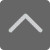## 爱智康资讯站

### 课程咨询: 4000-121-121

• 全国课程在线咨询
• 咨询热线：4000-121-121
• 登录手机官网

• 关注微信公众号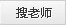# 期中数学备考知识初中数学公式：圆的标准方程及公式

2020-10-22 18:02:01 　来源：本站原创

## 学而思・爱智康1对1/8人班 帮助孩子解决知识难点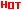*2470人已预约，首课体验不满意，可全额退费。

― ― 报名课程可获得 ― ―

圆的标准方程： (x-a)2+(y-b)2=r2 注：(a,b)是圆心坐标

圆的一般方程： x2+y2+Dx+Ey+F=0 注：D2+E2-4F>0

抛物线标准方程： y2=2px y2=-2px x2=2py x2=-2py

直棱柱侧面积： S=c*h 斜棱柱侧面积 S=c'*h

正棱锥侧面积： S=1/2c*h' 正棱台侧面积 S=1/2(c+c')h'

圆台侧面积： S=1/2(c+c')l=pi(R+r)l 球的表面积 S=4pi*r2

圆柱侧面积： S=c*h=2pi*h 圆锥侧面积 S=1/2*c*l=pi*r*l

弧长公式： l=a*r a是圆心角的弧度数r >0 扇形面积公式 s=1/2*l*r

锥体体积公式： V=1/3*S*H 圆锥体体积公式 V=1/3*pi*r2h

斜棱柱体积： V=S'L 注：其中,S'是直截面面积， L是侧棱长

柱体体积公式： V=s*h 圆柱体 V=pi*r2h

这里给同学们推荐数学期中真卷冲课程，希望同学们考试顺利--》点击查看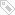标签：　期中数学备考知识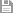保存　｜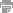打印　｜关闭